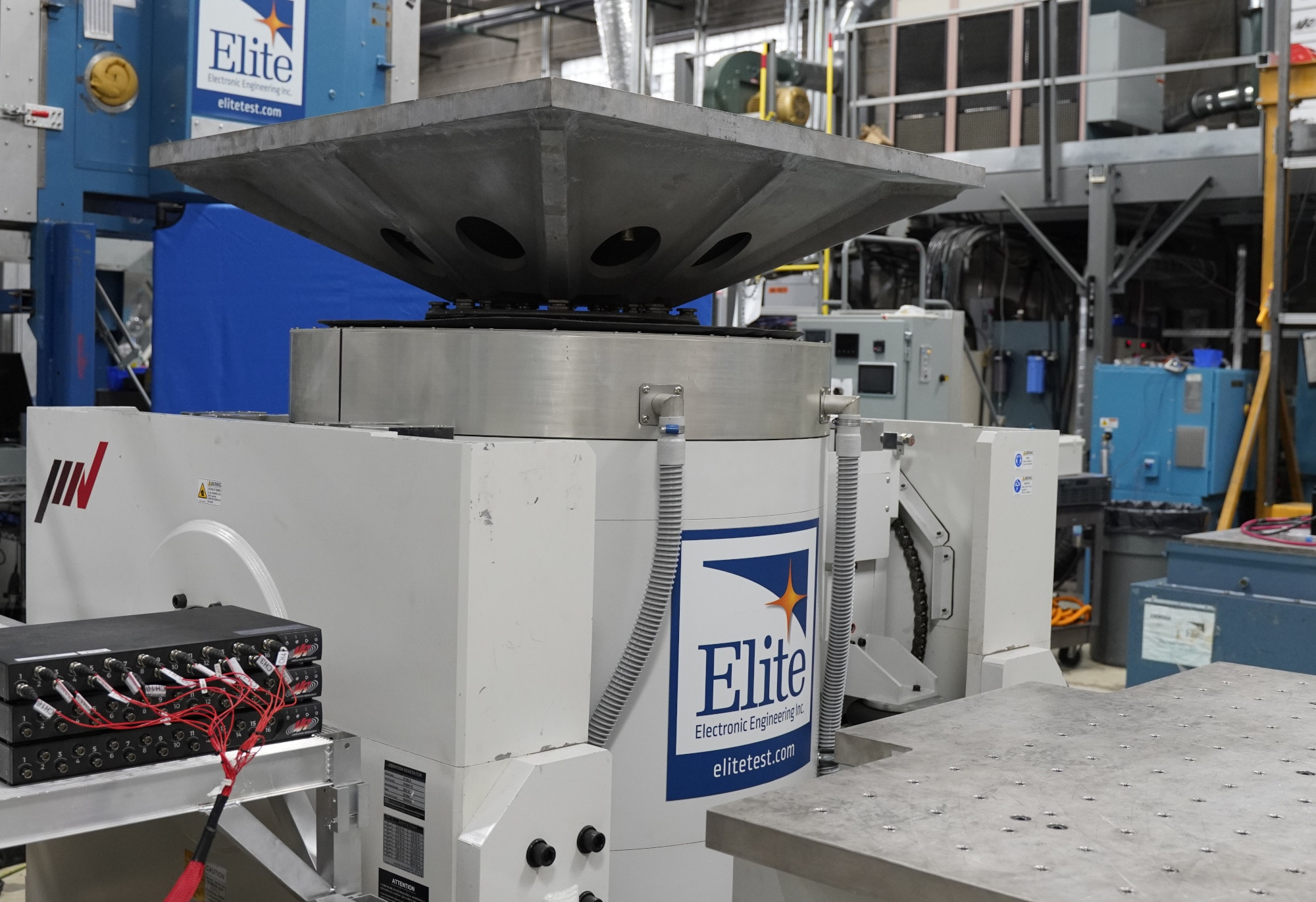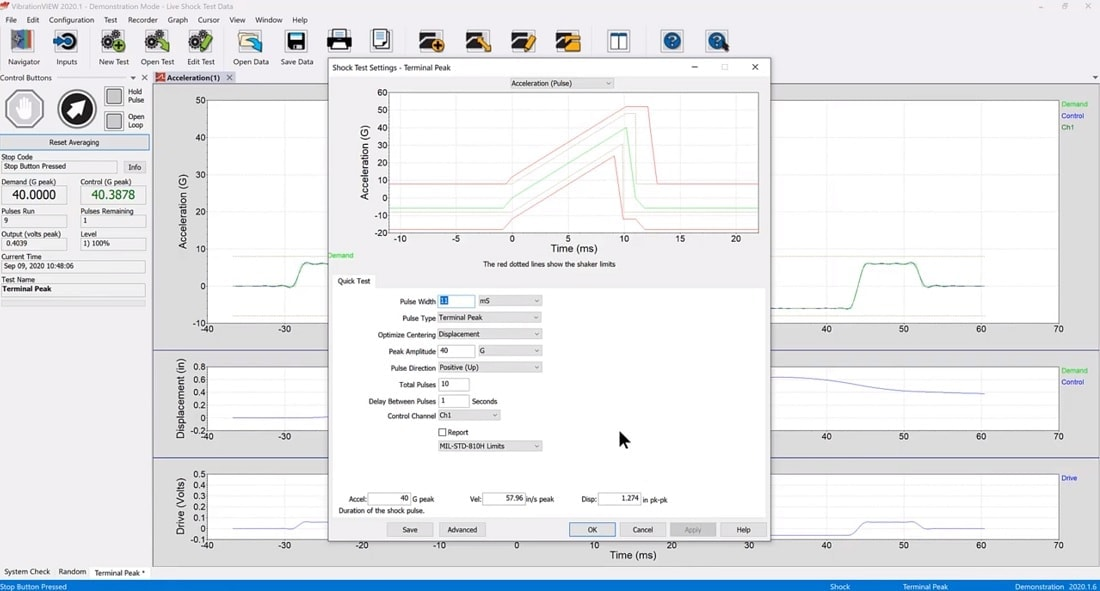Back to Articles

# Determining the Correct Shaker Size

#### Shakers

The equation Force = Mass x Acceleration is the basis for sizing shakers and determining if a shaker can perform a specific shock or vibration test. The more mass you put on your shaker, the less acceleration the shaker can produce. Conversely, the less mass you put on your shaker, the more acceleration it can produce.

### Shaker Force RatingsAll shakers have a force rating. These ratings determine what combination of mass and acceleration can be achieved on a given shaker. Yet, there are times where the maximum acceleration cannot be reached based on the shaker’s force rating and the calculated F=MA.

#### Example

For example, say you have a 500 force-lb shaker and want to perform a 5G sine test. The max product mass is 100lb (using M=F/A – 500 force-lb/5G). When the test is run, the shaker can only reach 4.75G.

In this case, it may seem that force doesn’t always equal mass times acceleration. Why is this? The most common reason is due to the weight of the armature inside the shaker. When you add the weight of the armature and any other moving mass (such as a driver bar when connecting a slip table to the armature), the maximum weight of your test item is reduced. The equation is correct, but all of the moving masses must be included.

The VibrationVIEW software performs these calculations automatically (download a free demo). The software includes many popular shaker models with their ratings pre-loaded for your convenience. Simply enter the total moving mass and VibrationVIEW will calculate the maximum acceleration for a given test type. The following are screenshots of the software calculating the same set of conditions as above:

The engineer enters the product mass (field 1) and armature mass (field 2), and the software calculates a total moving mass (field 3). With a shaker force of 500 force-lbs (field 4), the maximum acceleration is calculated (field 5). The maximum acceleration is less than 5G because the total moving mass is 105lbs. The engineer now must reduce the product mass or find a larger force shaker. If the engineer reduces the product mass to 95lbs, the desired acceleration of 5G can be achieved (fields 5 & 6).

### More on Shaker Limits

Shakers are designed to test products for shock and vibration and are sized based on the minimum expected level of moving mass. This is especially true for large force shakers. If we are testing a low mass product on a large force shaker and calculate the maximum acceleration level, the result is high maximum acceleration values.

#### Example

With a small product mass of 1lb (field 7), the total moving mass is 46lbs (field 8). Using a 30,000 force-lb shaker (field 9) the maximum calculated acceleration for sine testing is just over 650G. This is an incredibly high number for testing. The calculation is correct, but the shaker has limits on what acceleration levels it can perform at without damage. This is typically dependent on the physical characteristics of the shaker/armature and the voltage/current capabilities of the amplifier.

In this example, the “real” maximum acceleration level of the shaker (designated by the shaker manufacturer) should not exceed 125G. VibrationVIEW includes a field that allows the user to enter the maximum peak acceleration value, a sort of “never to exceed” value (fields 11 & 12).

### Other Shaker Limits

Although the focus here has been on calculating force, mass, and acceleration, shakers also have other limits for velocity, displacement (aka “stroke”), and drive voltage. VibrationVIEW includes limit fields for these parameters as well. Always double-check your shaker manual for the correct ratings. When in doubt, contact the shaker manufacturer for final clarification. After you have all of the correct ratings set up in VibrationVIEW, the software will display a warning message if you set up a test profile that is beyond your shaker’s capabilities.Date

December 8, 2020

Author

Jared Van Baren

Category